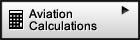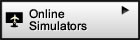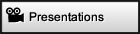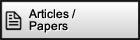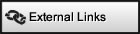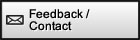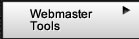If you would like to share,   e-mail or bookmark this   page, the tools below   are available for your   convenience.Surf with confidence!   Site has been tested by: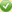Norton™ Safe WebMcAfee SiteAdvisor ®Article: Bank Angle for    Standard Rate Turn Your source for: Online Aviation Instrument Simulators + E6b, CR3 and other Flight Computer CalculatorsHome Page | 1 2 3 4 5 6 7 8 9 | Next

Bank Angle and the Physics of Standard Rate Turns (continued)

IV - Obtaining Approximate Equations for Mentally Estimating the
Bank Angle Required for Standard Rate Turn

Arctangent Approximation

Under certain conditions: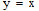is a very good approximation of: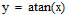Note that the atan (arctangent) function must be in radians.

Comparing Numerical Values for Arctangent

 x 0.000 0.087 0.176 0.268 0.364 0.466 0.577 0.700 y = atan (x) 0.000 0.087 0.174 0.262 0.349 0.436 0.523 0.611 y = x 0.000 0.087 0.176 0.268 0.364 0.466 0.577 0.700 Error (difference) 0.000 0.000 0.002 0.006 0.015 0.030 0.054 0.089 Error % 0% 0% 1% 2% 4% 7% 10% 15%

Table 4-1

Table 4-1 above shows several values for x and the corresponding y results. Decimals were placed to indicate the precision that the calculations were made. Notice that for values under 0.268 the difference is 2% or less.

 Arctangent (in Radians) Approximation and Error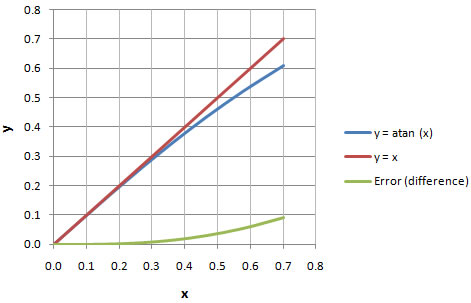Chart 4-1

In chart 4-1 you can visualize how the y = atan (x) function is very close to the straight line given by y = x for values closer to zero.

It is hard to see what this means for us when we are working in radians. The arctangent (atan) function in radians can be converted to degrees by simply multiplying it by a conversion constant c1.

Therefore we have: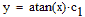where: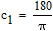and the simplified function will be: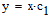where:Comparing Numerical Values for Arctangent
(in Degrees) with the Approximation

 x 0.000 0.087 0.176 0.268 0.364 0.466 0.577 0.700 y = atan (x) · c1 0.0 5.0 10.0 15.0 20.0 25.0 30.0 35.0 y = x · c1 0.0 5.0 10.1 15.4 20.9 26.7 33.1 40.1 Error (difference) 0.0 0.0 0.1 0.4 0.9 1.7 3.1 5.1 Error % 0% 0% 1% 2% 4% 7% 10% 15%

Table 4-2

Table 4-2 shows the values of y in degrees. For example when the value of the arctangent in degrees is 20, the simplified function will have a value of 20.9. This difference of 0.9 is approximately a 4% error. The higher the amount of degrees, the higher the error. For 35o the error is 15%. However for smaller angles the error is practically negligible.

 Arctangent (in Degrees) Approximation and Error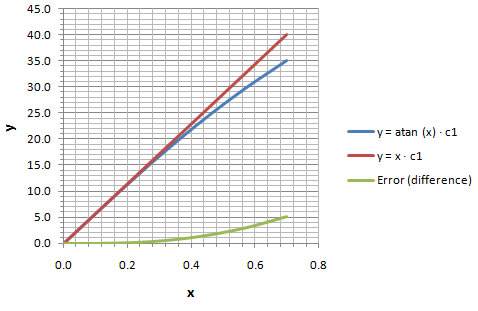Chart 4-2

Chart 4-2 helps visualize how the error is very minute for small angles and gets progressively larger once you pass about 25o.

Applying the Arctangent Approximation to our Formula for Bank Angle

Bank Angle = 57.296 · atan (0.0027467 · TAS)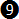c

Applying our simplification for arctangent, equationc becomes:

Bank Angle57.296 · 0.0027467 · TAS

Once again "" is just a reminder that this is an approximation. Now if we multiply the two numbers in our equation we will have:

Bank Angle0.15737 · TAS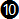Notice how remarkably close equationis to the rule of thumb formula discussed in the beginning of this article: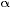0.15 · TAS

or:

Bank Angle0.15 · TAS

We had to go a long ways, but now we know where the rule of thumb formula comes from and what modifications had to be done to the exact formula in order to obtain it. Remember that Bank Angle in these two formulas is in degrees and TAS is in knots.

In summary we have the following (see table 4-3 below) equations for obtaining the bank angle for standard rate turn as a function of true airspeed (TAS):

Bank Angle Equations for Standard Rate Turn

 Equation Number Equation Exactc Bank Angle = 57.296 · atan (0.0027467 · TAS) yes*Bank Angle0.15737 · TAS no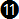Bank Angle0.15 · TAS noBank Angle0.10 · TAS + 5 no * Up to 5 significant digitsTable 4-3

The two rule of thumb equations that we discussed in the beginning of the article were numberedandso that we can refer to them later in the text. These are the equations that are easily mentally calculated because of their simplicity. In the next section we'll examine how precise they are.

 Home Page | 1 2 3 4 5 6 7 8 9 | Next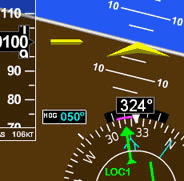Navigation Simulator Practice in our simulator specifically designed for learning the basics of instrument navigation. Try the Navigation Simulator, available for Windows. Free to try fully functional version for 10 days, \$39.95 to buy. Note: this simulator has its own self-contained Flash so it works without Flash being installed and is not subject to the Adobe Flash discontnuation / end of life.Free Online Simulators No installation required. These simulators are ready to run on your web browser and have a rich set of features. Practice basic VOR, ADF, RMI and HSI intrument orientation and execute holding patterns. Other simulators include pitot static system and altimeter errors. Click here to go to main online simulator page.All rights reserved to Luiz Roberto Monteiro de Oliveira.  No content or code of this web site may be reproduced without prior permission from the author.
LuizMonteiro LLC d/b/a luizmonteiro.com